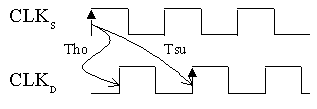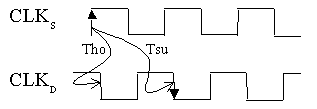# AR# 17224

|

## Description

When my design has a large positive skew, why do the rising-edge to rising-edge and falling-edge to falling-edge contain Hold violations, and my rising-edge to falling-edge and falling-edge to rising-edge do not? How are these calculations performed?

## Solution

The most important thing to note is that when a hold check is performed, it is checked with respect to the previous edge (the edge one period before the active edge). The diagram below shows what edges on the destination clock (Cold) are used to perform setup/hold checks.Note: Tsuji and Thom in the above diagram are not the actual setup/hold times of the flip-flops. They are simply referring to the edge that is used to calculate the setup/hold checks in the Xilinx tools.

You can observe in a register-to-register path with the same active edge (i.e., rising-edge to rising-edge) that a large positive clock skew greatly increases the chances for a hold violation (and, subsequently helps the setup calculation). See the timing diagram below for a rising-edge to falling-edge path.In this diagram, there is more positive clock skew than in the previous diagram, yet the window is still smaller, minimizing the chance for a hold violation. Consequently, a register-to-register path that has different clock phase (or different active edges) is less likely to have a hold violation and can handle more positive clock skew than a path that has the same clock phase (or same active edges).

In general, positive clock skew helps setup calculations. For this reason, the Xilinx tools truncate the positive clock skew to zero for setup calculations. In the diagrams above, the edge that Tsuji is referencing is the correct edge, but the actual calculation is performed earlier (without the positive clock skew).

The same theory applies for hold calculations. Since negative clock skew helps hold calculations, the Xilinx tools truncate the negative clock skew to zero for this calculation.
AR# 17224
Date 12/15/2012
Status Active
Type General Article
Tools
People Also Viewed